• 程序提供三个功能，一是进行方差分析，二是进行T计算，三是能解三元一次和二元一次方程组。单击对应的按钮即可进入对应的页面。是农业技术工作者不可多得的好工具。宽一点讲，凡是用得着以上功能的部门，都可能拿...
• 方差、协方差、标准(标准偏差/均方差）、均方误差、均方根误差(标准误差)、均方根 本文由博主经过查阅网上资料整理总结后编写，如存在错误或不恰当之处请留言以便更正，内容仅供大家参考学习。 方差...
  方差、协方差、标准差(标准偏差/均方差）、均方误差、均方根误差(标准误差)、均方根值

本文由博主经过查阅网上资料整理总结后编写，如存在错误或不恰当之处请留言以便更正，内容仅供大家参考学习。

方差（Variance)

方差用于衡量随机变量或一组数据的离散程度，方差在在统计描述和概率分布中有不同的定义和计算公式。①概率论中方差用来度量随机变量和其数学期望（即均值）之间的偏离程度；②统计中的方差(样本方差)是每个样本值与全体样本均值之差的平方值的平均数，代表每个变量与总体均值间的离散程度。

概率论中计算公式

离散型随机变量的数学期望：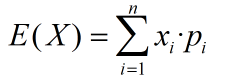---------求取期望值

连续型随机变量的数学期望：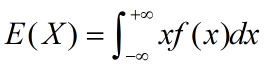----------求取期望值

其中，pi是变量，xi发生的概率，f(x)是概率密度。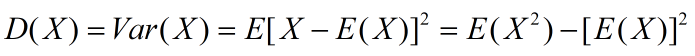---------求取方差值

统计学中计算公式

总体方差，也叫做有偏估计，其实就是我们从初高中就学到的那个标准定义的方差：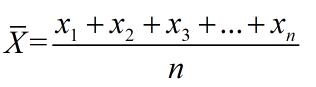-----------求取总体均值

其中，n表示这组数据个数，x1、x2、x3……xn表示这组数据具体数值。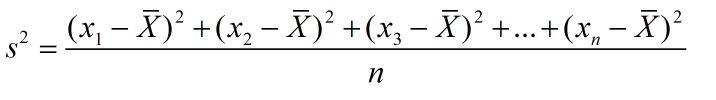------------求取总体方差

其中，为数据的平均数，n为数据的个数，为方差。

样本方差，无偏方差，在实际情况中，总体均值是很难得到的，往往通过抽样来计算，于是有样本方差，计算公式如下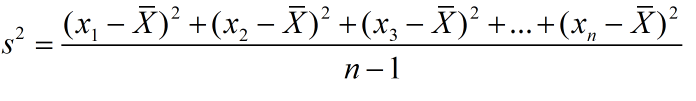--------------求取样本方差

此处，为什么要将分母由n变成n-1，主要是为了实现无偏估计减小误差，请阅读《为什么样本方差的分母是 n-1》。

协方差（Covariance）

协方差在概率论和统计学中用于衡量两个变量的总体误差。而方差是协方差的一种特殊情况，即当两个变量是相同的情况。协方差表示的是两个变量的总体的误差，这与只表示一个变量误差的方差不同。 如果两个变量的变化趋势一致，也就是说如果其中一个大于自身的期望值，另外一个也大于自身的期望值，那么两个变量之间的协方差就是正值。 如果两个变量的变化趋势相反，即其中一个大于自身的期望值，另外一个却小于自身的期望值，那么两个变量之间的协方差就是负值。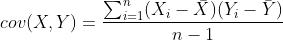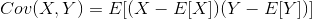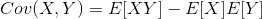其中，E[X]与E[Y]分别为两个实数随机变量X与Y的数学期望，Cov(X,Y)为X，Y的协方差。

标准差（Standard Deviation)

标准差也被称为标准偏差,在中文环境中又常称均方差，是数据偏离均值的平方和平均后的方根，用σ表示。标准差是方差的算术平方根。标准差能反映一个数据集的离散程度，只是由于方差出现了平方项造成量纲的倍数变化，无法直观反映出偏离程度，于是出现了标准差，标准偏差越小，这些值偏离平均值就越少，反之亦然。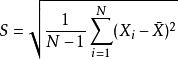------------求取样本标准差

其中，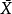代表所采用的样本X1,X2,...,Xn的均值。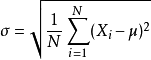-------------求取总体标准差

其中，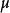代表总体X的均值。

例：有一组数字分别是200、50、100、200，求它们的样本标准偏差。= (200+50+100+200)/4 = 550/4 = 137.5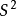= [(200-137.5)^2+(50-137.5)^2+(100-137.5)^2+(200-137.5)^2]/(4-1)

样本标准偏差 S = Sqrt(S^2)=75

均方误差（mean-square error, MSE）

均方误差是反映估计量与被估计量之间差异程度的一种度量，换句话说，参数估计值与参数真值之差的平方的期望值。MSE可以评价数据的变化程度，MSE的值越小，说明预测模型描述实验数据具有更好的精确度。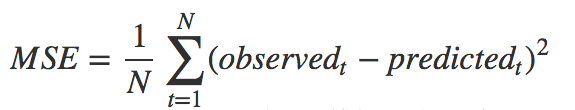均方根误差（root mean squared error，RMSE）

均方根误差亦称标准误差，是均方误差的算术平方根。换句话说，是观测值与真值(或模拟值)偏差(而不是观测值与其平均值之间的偏差)的平方与观测次数n比值的平方根，在实际测量中，观测次数n总是有限的，真值只能用最可信赖（最佳）值来代替。标准误差对一组测量中的特大或特小误差反映非常敏感，所以，标准误差能够很好地反映出测量的精密度。这正是标准误差在工程测量中广泛被采用的原因。因此，标准差是用来衡量一组数自身的离散程度，而均方根误差是用来衡量观测值同真值之间的偏差。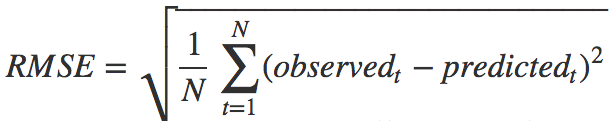均方根值（root-mean-square，RMES）

均方根值也称作为方均根值或有效值，在数据统计分析中，将所有值平方求和，求其均值，再开平方，就得到均方根值。在物理学中，我们常用均方根值来分析噪声。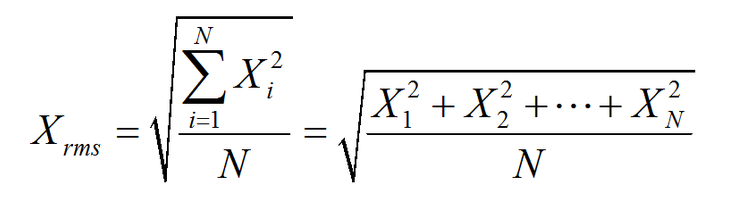比如幅度为100V而占空比为0.5的方波信号，如果按平均值计算，它的电压只有50V，而按均方根值计算则有70.71V。这是为什么呢？举一个例子，有一组100伏的电池组，每次供电10分钟之后停10分钟，也就是说占空比为一半。如果这组电池带动的是10Ω电阻，供电的10分钟产生10A 的电流和1000W的功率，停电时电流和功率为零。

展开全文• 文章目录什么是方差分析建立假设[^mcp]检验统计量偏差平方和均方F检验统计量使用Excel进行方差分析结果说明 什么是方差分析 Wikipedia: Analysis of variance (ANOVA) is a collection of statistical models used ...
文章目录什么是方差分析建立假设选择检验统计量偏差平方和$F$检验统计量给出拒绝域并做出判断使用Excel进行方差分析添加数据分析工具使用分析工具库结果说明

什么是方差分析

Wikipedia:
Analysis of variance (ANOVA) is a collection of statistical models used to analyze the differences among group means and their associated procedures (such as “variation” among and between groups), developed by statistician and evolutionary biologist Ronald Fisher.

方差分析（Analysis of variance，简称ANOVA，又称变异系数分析）是一类用于分析多组数据之间均值差异的统计方法模型，还涉及一些相关的步骤（比如两组数据之间的“变异”）。

从定义上看，方差分析是分析数据间均值的差异，那为什么叫“方差”分析，而不是“均值分析”？这是因为关于均值差异的结果是通过分析方差推算的，所以前者更合适。下面的具体步骤也将说明这一点。
这里仅对单因子方差方差分析1(one-way ANOVA)假设检验的基本步骤进行说明。
建立假设
设单因子$A$有$r$个水平，记为$A_1, A_2, ..., A_r$，不同水平($i$)下，各$m$次重复试验样本为$y_{ij}(i=1,2,...,r; j=1,2,...,m)$ ，每个水平可看作是一个总体，即水平$A_i$对应的$m$个观测样本集$\{y_{ij},j=1,2,....m\}$为一个总体，共$r$个总体。
假定，这里每个总体均为正态分布$N(\mu_i, \sigma_i^2)$，各总体方差($\sigma_i^2$)相等，所有试验样本之间相互独立。 建立如下一对假设：
$H_0:\mu_1=\mu_2=...=\mu_r \ \ \ vs \ \ \ H_1:\mu_1, \mu_2, ..., \mu_r$
若$H_0$成立，则说明因子$A$的$r$各水平间无显著差异，否则有显著差异。要检验原假设成立，即各总体均值相等，记总体均值为$\mu=(\sum_{i=1}^{i=r}\mu)/r$，第$i$水平的均值与总体均值$\mu$的差$a_i=\mu_i-\mu$作为$A_i$的水平效应，显然有$\sum{a_i}=0$，那原假设可看作是$H_0: a_1=a_2=...=a_r=0$，备择假设可看作是所以$a_i$不全为0。接着将给出检验$H_0$的统计量。
选择检验统计量
我们将通过偏差平方和建立检验统计量(F统计量)来对原假设($H_0$)进行检验。
首先，给出如下数据表：

因子水平
重复1
重复2
…
重复m

和
平均

$A_1$
$y_{11}$
$y_{12}$
…
$y_{1m}$

$T_1$
$\bar{y}_{1·}$

$A_2$
$y_{21}$
$y_{22}$
…
$y_{2m}$

$T_2$
$\bar{y}_{2·}$

…
…
…
…
…

…
…

$A_r$
$y_{r1}$
$y_{r2}$
…
$y_{rm}$

$T_r$
$\bar{y}_{r·}$

$T$
$\bar{y}$

其中，(令$i=1,2,…,r，n = r*m$)
\begin{aligned} T_i &= y_{i1} + y_{i2} + … + y_{im}, \\ \bar{y}_{i·} & = T_i / m, \\ T &= T_1 + T_2 + … + T_r, \\ \bar{y} &= (\bar{y}_1· + \bar{y}_2· + … + \bar{y}_r) / r \\ &=(T_1/m + T_2/m + … + T_r/m) / r \\ &=T / (r * m) \\ &=T/n. \end{aligned}
偏差平方和
一般在统计学中，$k$个数据$\{y_1,y_2,...,y_k\}$(记$\bar{y}$为该组数据的均值)，偏差平方和为$Q=\sum_{i=1}^{i=k}{(y_i-\bar{y})^2},$有时简称平方和。注意到，这$k$个偏差之和为$0$，即有如下恒等式：$\sum_{i=1}^{k=1}{(y_i-\bar{y})} = 0,$  这说明在$Q$中独立(线性无关)的偏差只有个$k-1$个， 在统计学中把$k-1$称为偏差平方和$Q$的自由度$df=k-1$。偏差平方和常用来度量一组数据的离散程度，对于上表中的数据，将计算如下几种偏差平方和：

总体偏差平方和$S_T$
这$n$个数据之间的差异大小用总体偏差平方和$S_T$表示: $S_T=\sum_{i=1}^{i=r}{\sum_{j=1}^{j=m}{(y_{ij}-\bar{y})^2}},$自由度为$\ df_T=n-1$，经推算可得：
\begin{aligned} S_T=\sum_{i=1}^{i=r}{\sum_{j=1}^{j=m}{y_{ij}^2}} - \frac{T^2}{n}. \end{aligned}

组内偏差平方和$S_e$
仅由随机误差引起各水平(组)内部间的误差，可用组内偏差平方和表示，也称误差偏差平方和：$S_e=\sum_{i=1}^{i=r}{\sum_{j=1}^{j=m}{(y_{ij}-\bar{y}_{i·})^2}},$ 自由度为$df_e=r(m-1)=n-r$.

组间偏差平方和$S_A$
组间除了随机误差，还有因子$A$各水平(各组)之间效应不同产生的差异，用组间偏差平方和表示（也称为因子$A$的偏差平方和): $S_A=m\sum_{i=1}^{i=r}{(\bar{y}_{i·}-\bar{y})^2},$自由度 $df_A=r-1$，经推算可得：$S_A=\frac{1}{m}\sum_{i=1}^r{T_i^2}-\frac{T^2}{n}$

从上述几种偏差平方和的表示方式很容易得到：$S_T=S_A+S_e,$ 自由度为$df_T=df_A+df_e$.
$F$检验统计量
根据上面偏差平方和的定义，样本均值间的差异可转化为样本偏差平方和的差异，那么要想知道各水平之间是否有差异，就可以通过组间差与组内偏差进行比较得出。（一般情况下，组间的样本多于组内样本，而样本越多偏差平方和也会越大）

若各水平间无差异，也就是各水平间只有随机误差，没有效应不同带来的误差，则组间偏差与组内偏差比较接近，也就是组间偏差与组内偏差的比值比较接近1；
若各水平间有差异，也就是各水平间除了随机误差，还有不同效应带来的误差，这时各水平间的误差大于组内误差，则组间偏差与组内偏差的比值会大于1。

那么这个比值达到什么水平，才认为两者之间有显著差异？也就是比值多大，才拒绝原假设？这需要选择一个合适的检验统计量。下面先引入均方的概念。
均方的定义为每个自由度上有多少个平方和，均方 $MS$表示为: $MS=\frac{Q}{df_Q},$其中，$Q$为偏差平方和，$df_Q$为对应偏差平方和的自由度。
由于均方的定义排除自由度不同所产生的干扰，这将有利于各组样本偏差平方和间的比较，所以将组间偏差平方和的均方与组内偏差平方和的均方的比值F作为检验$H_0$的统计量：
$F=\frac{MS_A}{MS_e}=\frac{S_A/df_A}{S_e/df_e}$
其中，$S_A, S_e$分别是组间偏差平方和与组内偏差平方和，$df_A, df_e$为对应的自由度。
给出拒绝域并做出判断
这里的检验统计量$F$就服从自由度为$df_A$和$df_e$的$F$分布，由于$F$的值越大，越倾向于拒绝原假设，因此该检验的拒绝域$W$为$W=\{F \geq F_{1-\alpha}(df_A, df_e)\},$ 其中，$\alpha$为显著性水平，一般取$0.05$，这里$F_{1-\alpha}(df_A, df_e)$通过$F$分布查表获得。也就是说，

若$F \geq F_{1-\alpha}(df_A,df_e)$，则因子$A$各组间有显著差异；
若$F < F_{1-\alpha}(df_A,df_e)$，则说明因子$A$各组间差异不显著。

该检验的$p$值通过$F$分布对应的密度函数得到:$p=P(Y \geq F).$
$p$值是利用样本观测值做出拒绝原假设的最小显著性水平，一般在实际中，若$p$值很小($p \leq 0.001$)时，可做出拒绝原假设；若$p>0.05$时，接受原假设。当$\alpha$与$p$比较接近时，才比较$\alpha$和$p$。如果$\alpha \geq p$，则在显著性水平$\alpha$下拒绝$H_0$；否则，在显著水平$\alpha$下接受原假设。

关于数据的计算可使用统计分析工具，一般有SPSS, SAS等，当然还有一般人接触最多的Excel，下面是利用Excel中的分析工具进行单因子方差分析。
使用Excel进行方差分析
添加数据分析工具
若功能区已经显示有“数据分析”功能（如下图）可跳过下面三步选择“数据”-> 右键“数据工具”-> 选择“自定义快速访问工具栏”，选择后将弹出“Excel选项”窗口(也可通过其他方式打开)；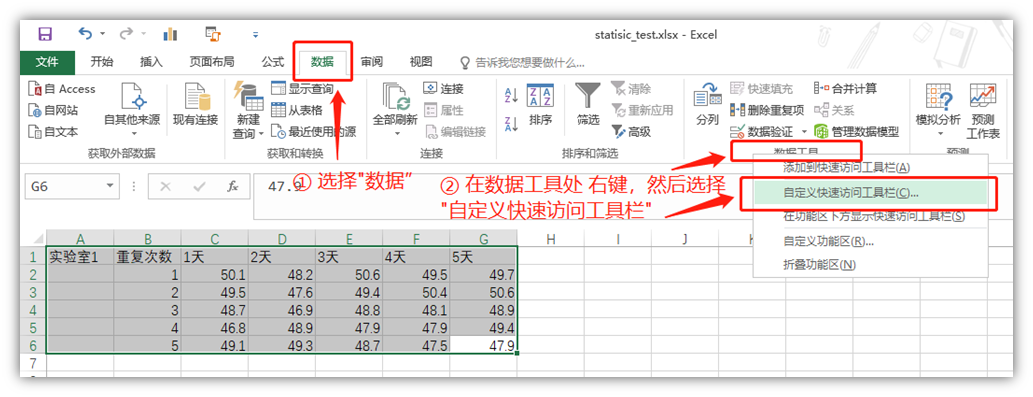“Excel选项” -> “加载项” -> “分析工具库-VBA”-> “转到”，选择后会弹出“加载宏”的窗口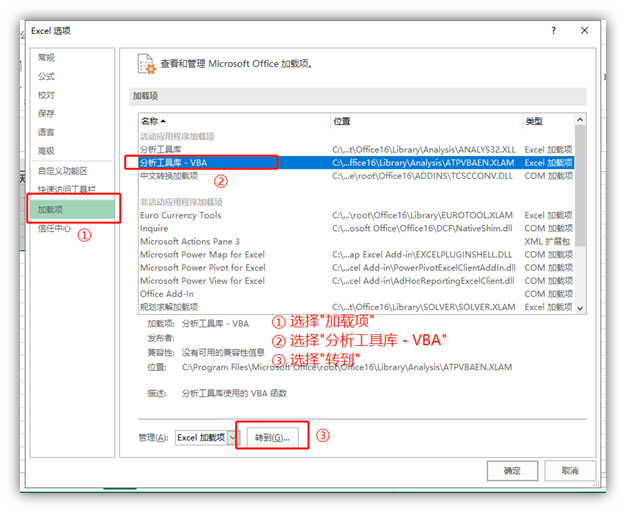“加载宏”-> 在“分析工具库 - VBA”前打对勾 -> “确定”，此时Excel上的功能区就会显示“数据分析”工具(如最上面第一张图)使用分析工具库
使用如下数据进行示例操作：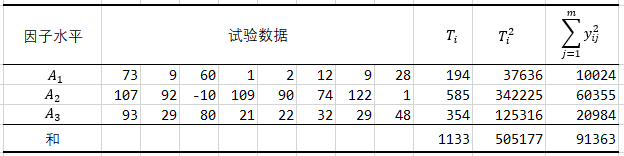可根据上面方差分析的步骤直接计算，下面是使用Excel中的数据工具直接得到偏差平方和，自由度，均方等。
使用分析工具库进行单因子方差分析步骤：

“数据分析”-> “方差分析：单因素方差分析”-> 点击“确定”后，会出现方差分析选项卡。这里请注意，Excel分析工具进行方差分析时，一列作为一组，所以将上面数据进行转置。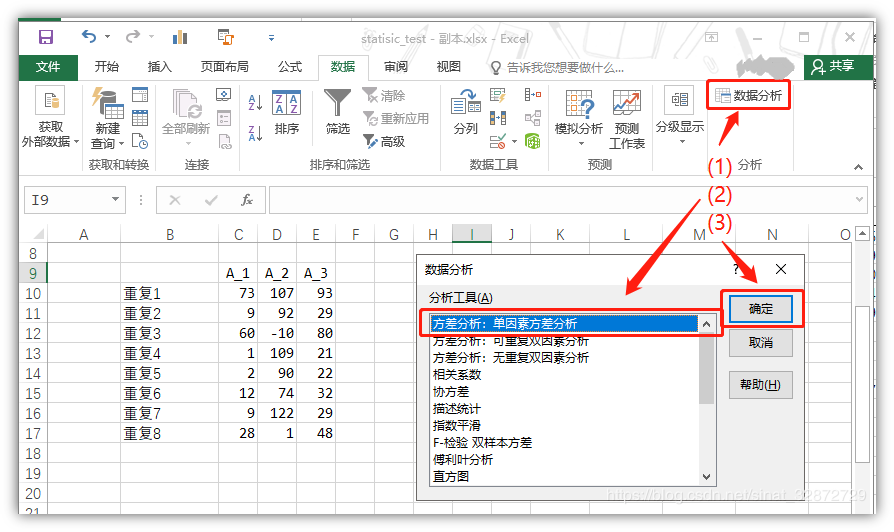“方差分析：单因素方差分析”选项卡 -> 在“输入区域”选择要分析的数据(可通过右侧按钮鼠标选择，也可直接手动输入) -> 在“$α$(A)”的选择填显著性水平(默认0.05) -> 在“输出区域”选择输出单元格 -> 点击“确定”后，会计算出对应数据的结果，其中包含平方和(SS) 、自由度(df)，均方(MS) 等。(注：选项卡中"标志位于第一行"勾选表示 数据第一行为列名，在输出结果时会显示对应列名，若数据未选择列明则无需勾选，结果中列名会对应写成"列1", “列2”,…)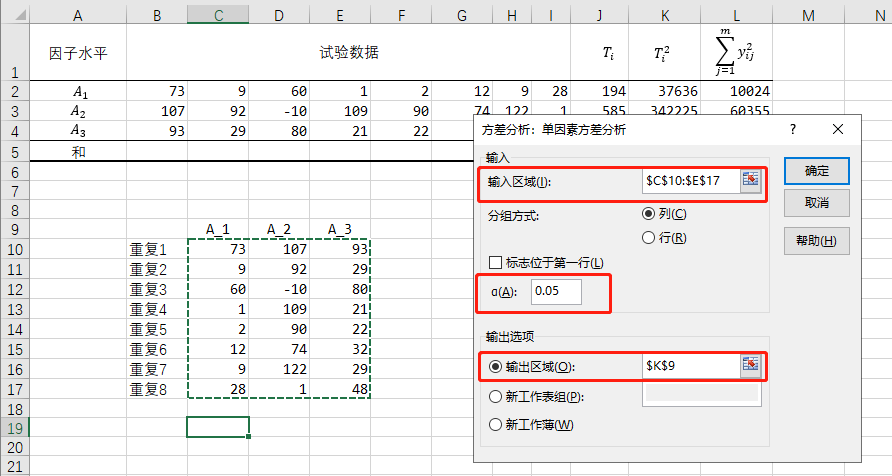点击确定后，就会直接给出该数据对应的方差分析表：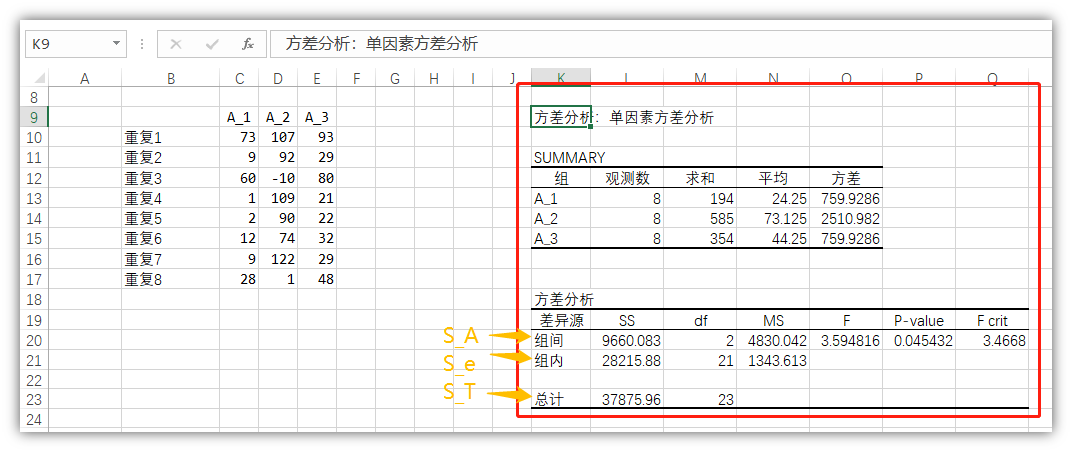结果说明
结果中给出了两个汇总表：

第一个是各组数据之和，平均值，方差；
第二个是方差分析的结果表，其中
- $SS$ 表示偏差平方和
- $df$ 表示自由度
- $MS$表示均方
- $F$是对应组间和组内偏差平方和均方比值
- $P$-$value$为对应$F$值的密度函数值，也可利用Excel中=F.DIST.RT(<F值>,<组间自由度>,<组内自由度>)\$公式得到
- $F crit$为对应$F$分布，$\alpha$置信水平下自由度为$df_{组间}$和$df_{组内}$的临界值，也可利用Excel中=F.INV(1-<\alpha>,<组间自由度>,<组内自由度>)公式得到

茆师松,程依明, 濮晓龙.概率论与数理统计教程第二版[M].高等教育出版社:北京,2011:423-434. ↩︎


展开全文Excel
• 方差分析(Analysis of Variance，简称ANOVA) ...每一个F值都会对应一个p值，F值越大，p值越小 所以实际上F值和p值都能用来检验显著性差异 p-value就是用于检验特征与变量之间相关性的 假设你给出α值（常常取0.05，0.01
方差分析(Analysis of Variance，简称ANOVA)
用于两个及两个以上样本均数差别的显著性检验。
作用：确定参数对算法性能的影响
基础概念：方差分析
如何理解p值和F值

Sum.sq.——平方和
d.f.	——自由度
Mean.Sq.——均方
F-value——F值
p-value——p值

关于F值和p值
每一个F值都会对应一个p值，F值越大，p值越小
所以实际上F值和p值都能用来检验显著性差异
p-value就是用于检验特征与变量之间相关性的
假设你给出α值（常常取0.05，0.01），如果你的p-value小于α，那就有把握认为，这个特征和预测变量y之间，具有相关性。
假设：H0——无差异；H1——有显著差异
P>0.05称“不显著”；P<=0.05称“显著”，P<=0.01称“非常显著”。
P>0.05只能说明没有充分的证据说明二者确有差别，但是也不能说二者没有差别或差别很小。

F是显著性差异的水平，用计算出的F值与F表中的值对比，就可以确定是否存在显著性差异。
F < F表 表明两组数据没有显著差异；
F ≥ F表 表明两组数据存在显著差异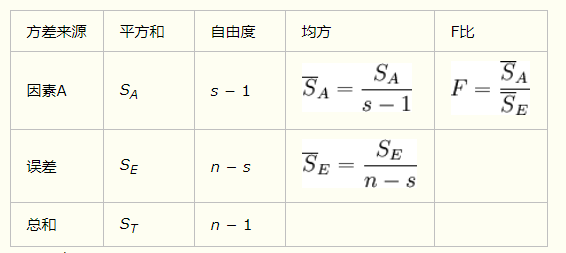展开全文• 从形式上看，方差分析与之前的t检验或z检验区别不大，都是检验均值是否相等，但在比较多个均值时，t检验需要做多次两两比较的假设检验，而方差分析只需要一次，并且方差分析中是将所有的样本信息结合在一起，增加了...
第四部分的卡方检验是研究类别变量之间的关系，而这一部分的方差分析则是研究类别型自变量与数值型因变量之间的关系，它在形式上是比较多个总体的均值是否相等。
从形式上看，方差分析与之前的t检验或z检验区别不大，都是检验均值是否相等，但在比较多个均值时，t检验需要做多次两两比较的假设检验，而方差分析只需要一次，并且方差分析中是将所有的样本信息结合在一起，增加了分析的可靠性。
下面我们仍旧从三个问题出发来研究一下方差分析：

方差分析到底是啥？
方差分析从哪里来？
方差分析要到哪里去？（有什么用？）

这里并不打算按照这个顺序来分析这三个问题，因为很多理论都是为了解决某些实际问题而提出的，理论只是对解决方法的高度概括，从实际问题出发，反而可以更好地理解为什么要研究这些理论。下面将从以下几个方面来回答这些问题：

1、为什么要做方差分析？（方差分析有什么用？）
2、方差分析到底是啥？从哪里来的？
3、方差分析的基本假定
4、单因素方差分析

1、为什么要做方差分析？
这里举个实际的例子来引出方差分析，这样会对方差分析具体在做什么有更深的印象：
为了研究客户满意度是否与行业有关，消费者协会对各个行业抽样了数量不等的公司，分别统计了各个公司的被投诉次数：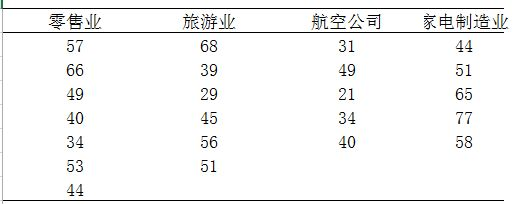那么基于以上的数据，你是否可以判断客户的投诉次数与行业有关。
问题其实比较清晰，就是比较这四个行业之间被投诉的均值是否相等，这里的行业就是类别型自变量，被投诉次数就是数值型因变量。所以方差分析要研究的就是行业对被投诉次数是否有显著影响。
2、方差分析到底是啥？从哪里来的？
方差分析的思想来自于误差的分解，对于来自同一个分布的数据，抽样带来的误差其实只有随机误差，即随机抽样抽到的不同值与均值之间的差距，以上面这个例子为例，我们可以把各个行业内部的抽样数据认为是来自同一个分布，即零售业不同企业有不同的被投诉次数，他们之间的不同可以认为只是随机误差，也被称为组内误差（SSE）。
不同行业之间误差被称为组间误差(SSA)，如果被投诉次数与行业无关，可以认为不同行业的抽样也是来自于同一总体分布的抽样，那么此时误差只有随机误差。而如果不同行业的抽样并不是来自同一总体分布，意味着被投诉次数与行业有关，那么此时组间误差包括随机误差和系统误差，这个系统误差就是由于行业这个因素带来。
综合以上，我们可以将总体误差（SST）分为组间误差和组内误差。
当我们检验不同行业的均值是否相等时，其实就是检验组间误差是不是过大，包含了一定量的系统误差。
3、方差分析的基本假定
有三个基本假定：
每个总体都应服从正态分布；
每个总体的方差必须相同；
观测值是独立的；
其中第三点一般都满足，前两点需要借助样本值做一些检验的工作；
4、单因素方差分析（F检验）
如果只涉及一个类别的自变量的方差分析，则称其为单因素方差分析，最上面的例子就是一个单因素方差分析。
首先提出假设：
$H_0 = \mu_1 = \mu_2 = ... = \mu_k$
$H_1 = \mu_i (i = 1...k)$不全相等
构造统计量：
1) 计算个样本的均值
即计算各个行业的均值：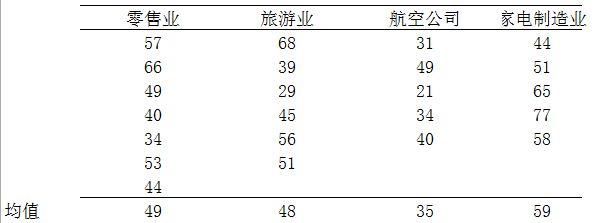2) 计算总均值
即计算总体均值：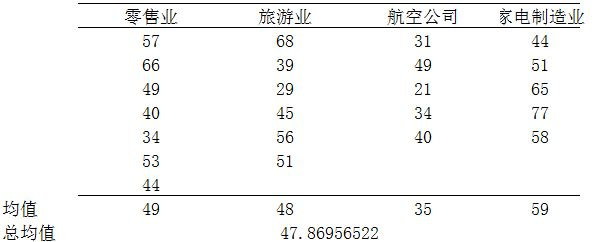这里：
$\overline {\overline X} = {57 + 66 + ... + 77 + 58 \over 23} = 47.87$
$\overline X_1 = 49 \overline X_2 = 48, \overline X_3 = 35, \overline X_4 = 59$
求这四个平均值的平均值同样得到47.87。
所以，总均值可以看成所有数据点的均值，或每组均值的均值。

3) 计算各误差平方和

总平方和：

$SST = \sum^k_{i=1}\sum^{n_i}_{j=1}(x_{ij} - \overline {\overline x})^2 = (57 - 47.869)^2 + ... + (58 - 47.47.869)^2 = 4164.608$

组间平方和：

$SSA = \sum^k_{i=1}(\overline x_{i} - \overline {\overline x})^2 = 7*(49 - 47.869)^2 + ... + 5*(59 - 47.869)^2 = 1456.608$

组内平方差：

$SSE = \sum^k_{i=1}\sum^{n_i}_{j=1}(x_{ij} - \overline x_i)^2 = (57 - 49)^2 + ... + (58 - 59)^2 = 2708$
并且：$SST = SSA + SSE$
4) 计算统计量
SST的自由度为n-1，n为观测值的个数；
SSA的自由度为k-1，k为因素水平的个数；
SSE的自由度为n-k。

平方和
自由度
自由度计算公式

SST= 4164.608
22
n - 1

SSA = 1456.608
3
k - 1

SSE = 2708
19
n - k

故：
总平方和 = 组内平方和 + 组间平方和
我们其实是要比较SSA与SSE，因为我们确认SSE，即组内误差来自随机误差，那我们就以SSE为基准，去比较SSA，若二者相差不大，则认为组间误差也仅仅是随机误差而没有系统误差，而由于二者之间的数量级不一样（自由度），难以直接比较，所以我们可以比较二者的均方误差：
组间均方误差：$MSA = {SSA \over k-1}$ 服从 $\chi^2(k-1)$
组内均方误差： $MSE = {SSE \over n-k}$ 服从 $\chi^2(n-k)$
于是，我们构造$F$统计量：$F = {MSA \over MSE} \sim F(k-1, n-k)$
计算可得统计量的值为：
$F = 3.406$

这里定义的统计量叫F统计量，它服从F分布，这里可以认为它是两个$\chi^2$分布之比，两者的自由度可以相等，也可以不等。
$\;$
F统计量是组间平方和除以其自由度k-1，也被称为组间均方误差（MSA），然后除以组内平方和除以其自由度n-k：
$F = {MSA \over MSE} = {{SSA \over k-1} \over {SSE \over n-k}}$
如果分子比分母大很多，那就说明波动大多数来自于各组之间，较少来自于各组之内，这时我们应该相信，总体均值之间存在差异，如果这个数字很大，那就意味着零假设成立的概率较低；
如果这个数字很小，分母更大，那就意味着组内波动比组间波动在总波动中占比更多，这意味着差异可能只是随机产生的，这就更难拒绝零假设。

对于这个问题，$\alpha = 0.05, n_1 = 3, n_2 = 19$，从下面的F分布表中可以读出临界F值：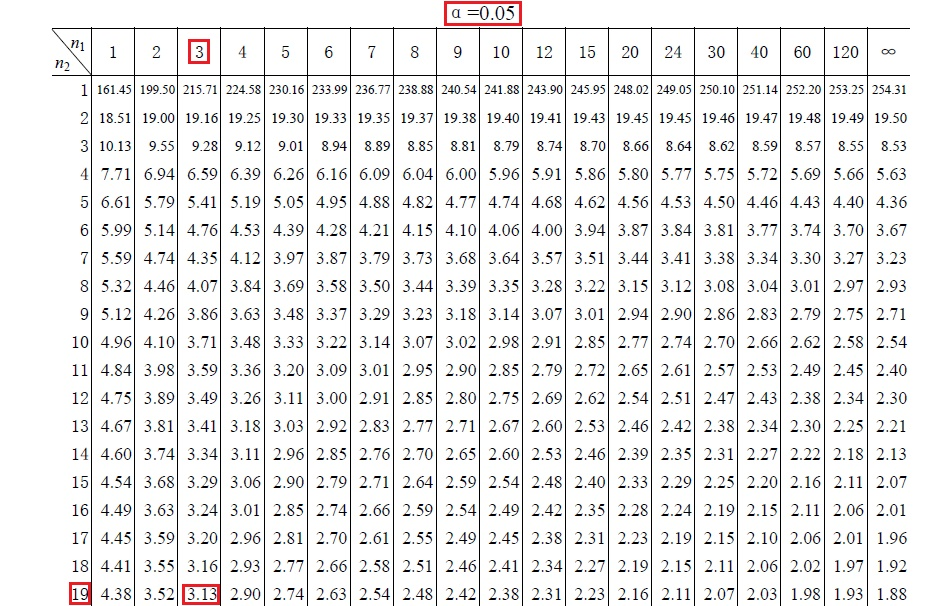这里从F分布统计表中读出的临界F值是：
$F_c = F_{0.05}(3, 19) = 3.13$
因为$F = 3.406 > F_c = 3.13$，所以，在零假设前提下，得到观测值的概率非常低，因此我们拒绝零假设，相信很有可能总体均值存在差异。
参考文献：
 方差分析
 统计 可汗学院


展开全文• 前面我们说了方差分析中的单因素方差分析，但是在实际工作中，影响因素往往不止一个，需要考虑两个或以上因素对实验结果的影响。比如某公司销售汽车，在销售时，除了要关注汽车的外形、价格、耗油量等因素以外，还要...数据挖掘 机器学习 算法
• 2020年初，整个世界遭受了新冠病毒地袭击，直到今天人类还没有走出阴霾。抗疫前线的医学专家们日以继夜地工作，同时进行着多种药物的临床试验。那么怎么判断哪一种药物效果更好呢？...本文下面要讲到的方差分析F
• 双因素方差分析 1. 双因素方差分析的理论 2. 双因素方差分析的实现python
• SPSS分析基础——方差分析单因素ANOVA分析数据介绍数据分析单变量方差分析数据介绍（grocery_1month.sav）数据...方差分析(Analysis of Variance，简称ANOVA)，又称“变异数分析”或**“F检验”，用于两个及两个以...
• ## Matlab方差分析

万次阅读 多人点赞 2018-11-01 19:57:33
Matlab 方差分析（T检验） 在工农业生产和科学研究中,经常遇到这样的问题:影响产品产量、质量的因素很多,我们需要了解在这众多的因素中,哪些因素对影响产品产量、质量有显著影响.为此,要先做试验,然后对测试的结果...T检验
• 检验统计的设定和检验方法与变量间的方差是否相等有关。 例如研究行业、服务等级对投诉数的影响：如表格中给出4个行业、每个行业有3个服务等级、样本容量为7、观测为投诉数。则构成一个3维的矩阵。 在上述基础上...
• ## SPSS篇—方差分析

万次阅读 多人点赞 2019-08-20 14:03:23
方差分析又被称作“F检验”或者“变异数分析”，主要是用于两个及两个以上样本均值差异的显著性检验。方差分析和回归分析一样，也有很多个分支。对于方差分析，一般我们是用来研究不同来源的变异对总变异的贡献大小...
• 无论是什么活动，影响产品质量和产品的因素都有多种，如影响农作物产量的因素有品种、天气、施肥量、肥料的种类等等。如果我们想要了解这些因素中哪些因素对产量有显著性影响，或各个...方差分析(Analysis of Varia...机器学习 数据挖掘 算法
• 之前介绍了单因素方差分析，对双因素方差分析有相似，也有差异。下面是对双因素方差分析作简单介绍。 自由度推算： https://doc.mbalib.com/view/2e390b8fd8b8410554639a1fb590e1e8.html ...
• 当我们想了解不同年级的学习态度是否有区别，进而提供有...方差分析用于定类数据（X）与定量数据（Y）之间的差异分析，例如研究三组学生（X）的智商平均（Y）是否有显著差异。其中X的组别数量至少为2，也可以分...
• 方差分析基本原理样本要求 独立性 各样本必须是相互独立的随机样本 样本含量尽可能相等或相差不大 可比性 样本均值不相同，可比较 正态性 样本的总体符合正态分布，偏态分布不适用于方差分析。 对偏态分布应......